Code available here

## Problem

Classifying videos with deep learning architectures is hard

#### CNN only

Two options:

1. Big 3D volumes with variable sizes that might not fit into memory.
2. Process each frame separately and fuse the results to make a prediction

#### CNN+RNN

Using CNN+RNN with end-to-end training is impractical. In practice, 2 options were explored :

1. Focus on the CNN part and reduce the sequence length
2. Focus on the RNN part and use a pre-trained CNN (no end-to-end training)

#### RNN only

Using only an RNN is also a problem, given the input dimensionality (e.g. a video with $$160 \times 120 \times 3$$ frames results in a $$57,600$$ input vector, and $$5,760,000$$ parameters if using a 100-units hidden layer).

## Summary

The paper focuses on RNN-only video classification using raw pixels. To reduce the input dimensionality, the input-to-hidden matrix is factorized with the Tensor-Train decomposition.

### Tensor-Train Factorization

#### Step 1: Factorization

Each entry in the target tensor is represented as a sequence of matrix multiplications using elements selected from core-tensors.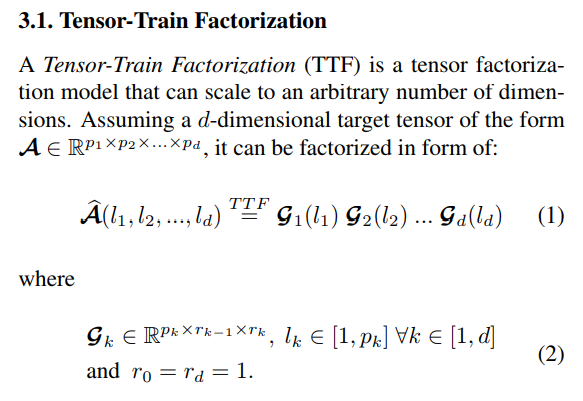Each index $$l_k$$ is used to select an element from the core-tensors along the first dimension $$p_k$$. Note that since $$r_0 = r_d = 1$$, the result is always a scalar.

Visually :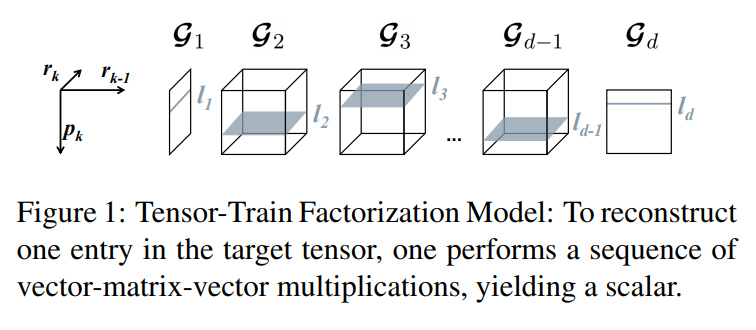#### Step 2: Preliminary steps for Feed-Forward layer factorization

We add the constraint that each integer $$p_k$$ can be factorized as $$p_k = m_k \cdot n_k \, \forall \, k \in [1,d]$$. Then, we reshape $$\mathcal{G}_k$$ into $$\mathcal{G}_k^* \in \mathbb{R}^{m_k \times n_k \times r_{k-1} \times r_k}$$. Each index $$l_k$$ can be uniquely represented with two indices $$(i_k, j_k)$$: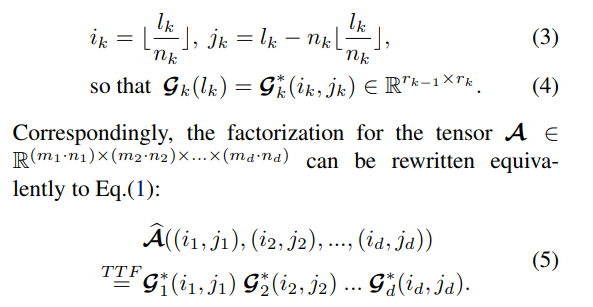#### Step 3: Feed-Forward Layer Factorization

The usual notation for a feed-forward layer is: $$\hat{\mathbf{y}} = \mathbf{Wx} + \mathbf{b}$$ The equation is rewritten in an equivalent way using scalars: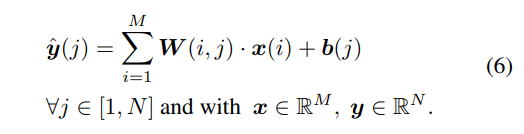If both $$M$$ and $$N$$ can be factorized into integer arrays of length $$d$$, i.e. $$M = \prod_{k=1}^d m_k, N = \prod_{k=1}^d n_k$$, then $$\mathbf{x}$$ and $$\hat{\mathbf{y}}$$ can be reshaped to have the same number of dimension: $$\mathcal{X} \in \mathbb{R}^{m_1 \times ... \times m_d}$$, $$\mathcal{Y} \in \mathbb{R}^{n_1 \times ... \times n_d}$$. The mapping function $$\mathbb{R}^{m_1 \times ... \times m_d} \rightarrow \mathbb{R}^{n_1 \times ... \times n_d}$$ is rewritten as: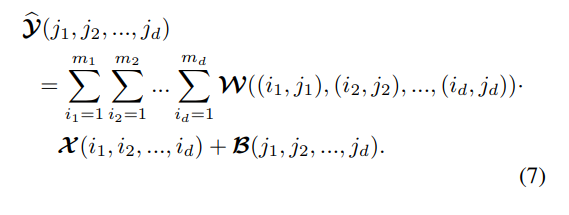Thus, Eq.6 is a special case of Eq.7 with $$d = 1$$. Finally, the weight tensor $$\mathcal{W}$$ can be replaced by the TTF representation: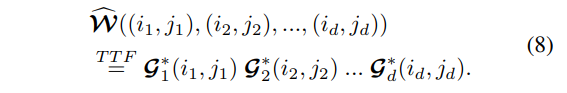#### Step 4: Tensor-Train RNN

The LSTM has 4 “gates”, or weights matrices. Each of those matrices can have a TTF representation. However, a concatenation trick can be used like most LSTM implementations. Thus, all gates are concatenated as one big output tensor which can be compressed even more.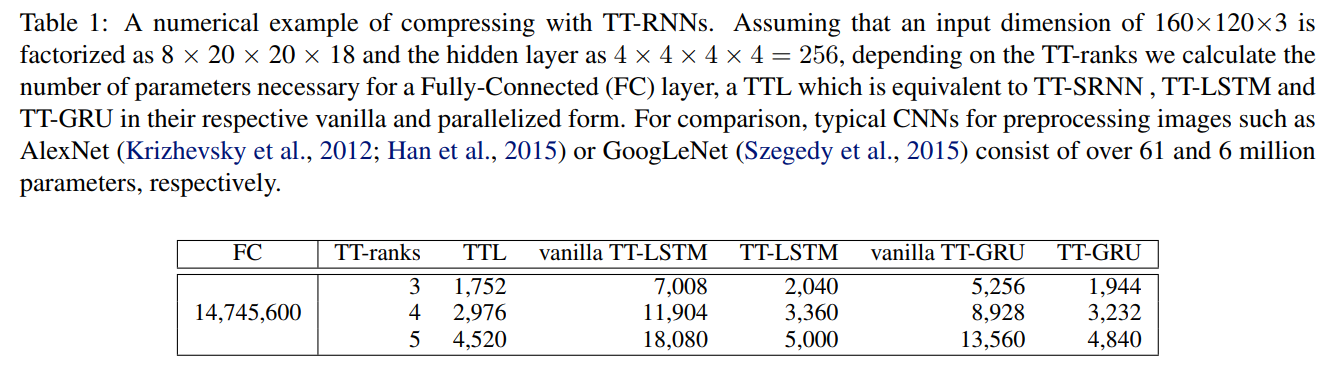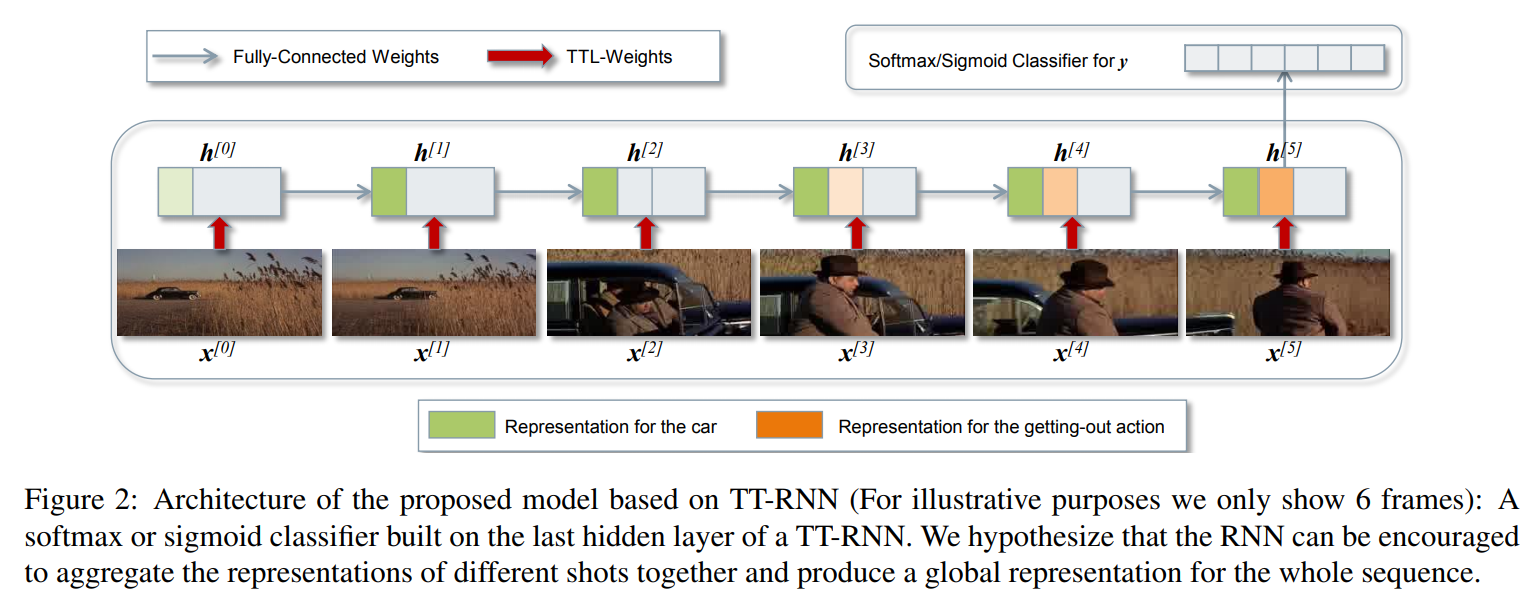## Experiments

• 1600 video clips
• 11 classes
• $$320 \times 240$$ downsampled to $$160 \times 120$$, at 24 fps
• Sequence length varies between 204 and 1492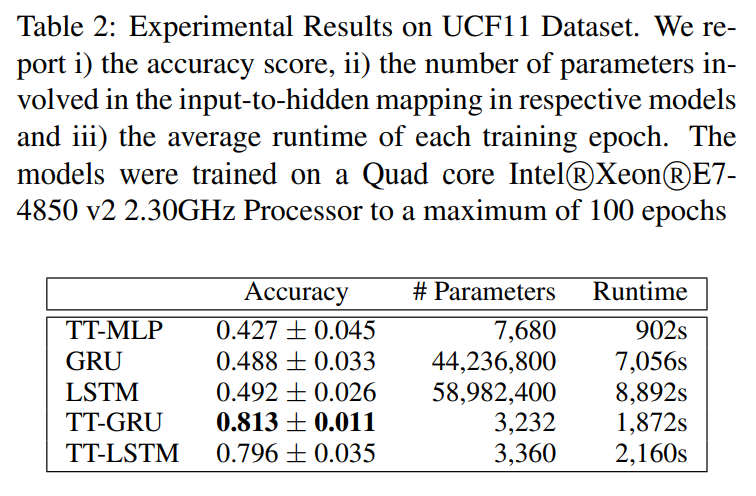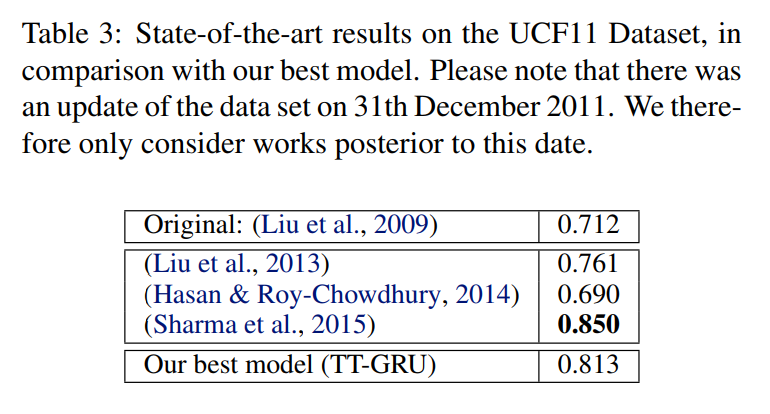• (Liu et al., 2013): SIFT/STIP + Harris3D Corner Detector + Optical Flow + GMM w/ EM
• (Hasan & Roy-Chowdhury, 2014): Ensemble of multi-class SVMs
• (Sharma et al., 2015): Pre-trained GoogLeNet CNN + 3-fold stacked LSTM + Attention

Training times: 8-10 days for GRU/LSTM vs. 2 days fro TT versions.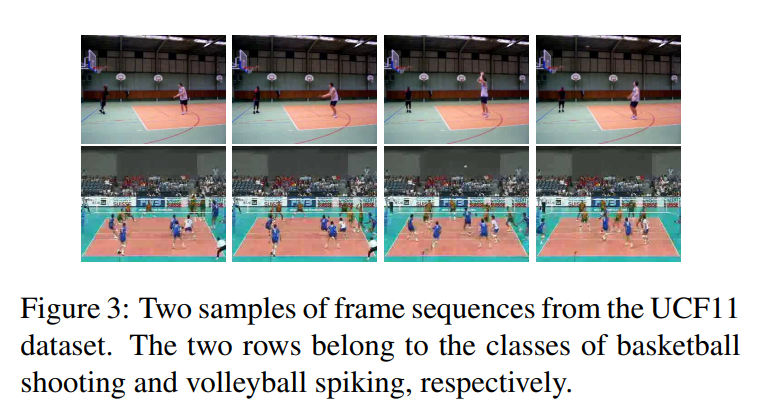### Hollywood2 Data

• 69 movies (33 training, 36 test) -> 823 training clips, 884 test clips
• 12 labels, multiclass
• $$234 \times 100$$, at 12 fps
• Sequence length varies between 29 and 1079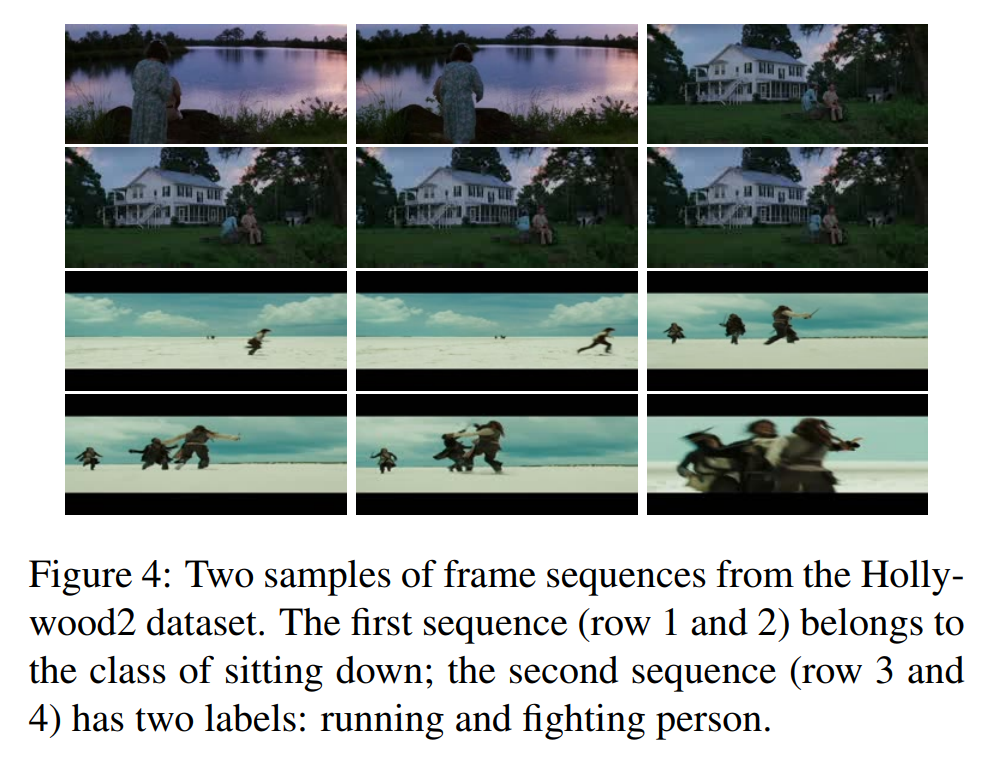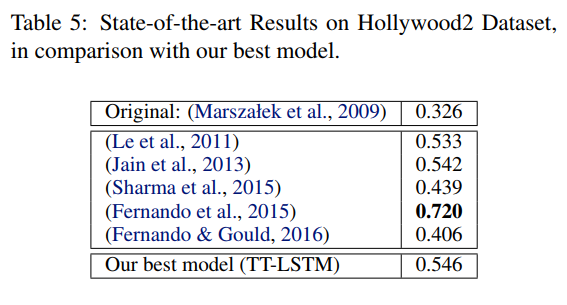• (Sharma et al., 2015): Pre-trained GoogLeNet CNN + 3-fold stacked LSTM + Attention
• (Fernando et al., 2015): Frame features + Frame ordering -> Learned representation -> SVM classifier
• (Fernando & Gould, 2016): Frame-wise CNN + “Rank pooling” + Softmax